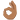# If supremum=infimum, is f Riemann integrable?

This is my first time posting & I am not familiar with how to get all the correct math symbols or how to use Latex, so I did the best I could.

## Homework Statement

Suppose f is bounded on [a,b] and there is a partition P* of [a,b] for which S(f,P*)=S(f,P*). Is f Riemann integrable on [a,b]?

## Homework Equations

S(f)=sup{S(f,P*): P* is partition of [a,b]}
S(f)=inf{S(f,P*); P* is partition of [a,b]}

## The Attempt at a Solution

I know, by a theorem, that S(f)>S(f). I am trying to figure out how to show S(f)<S(f) so that I can say S(f)=S(f). I thought about choosing another partition Pe such that S(f,Pe)-S(f,Pe) would equal some epsilon value, but I don't know what value I should use or where to go next.

If this is the wrong process for this proof, I would love a hint on where to start.

## The Attempt at a Solution

Last edited:

Did you mean "for which S(f, P*)=S(f)"? If so, hint: consider characteristic function of rationals on [0,1].

Did you mean "for which S(f, P*)=S(f)"? If so, hint: consider characteristic function of rationals on [0,1].

No, I am trying to use a theorem that says a function is Riemann integrable if the supremum equals the infimimum: S(f)=S(f)

Are you using Darboux definition of Riemann integral, I mean:
$$\underline{S}(f, P^*)=\sum m_i \Delta x_i$$
$$\overline{S}(f, P^*)=\sum M_i \Delta x_i$$
where
$$m_i = \inf \{f(x): x_{i-1}\leq x \leq x_i\}$$
$$M_i = \sup \{f(x): x_{i-1}\leq x \leq x_i\}$$
or the other popular one, where
$$S(f, P^*)=\sum f(t_i)\Delta x_i$$
for $$x_{i-1}\leq t_i \leq x_i$$
?
Sorry for such question, but I'm confused. Statement like "S(f, P*)=S(f, P*)" suggests the first (Darboux) definition, while "Relevant Equations" section suggests the second (at least for me). Or maybe it's yet another definition? Again, sorry to ask, but when I find out which definition you are using, I think I will be able to help.

Are you using Darboux definition of Riemann integral, I mean:
$$\underline{S}(f, P^*)=\sum m_i \Delta x_i$$
$$\overline{S}(f, P^*)=\sum M_i \Delta x_i$$
where
$$m_i = \inf \{f(x): x_{i-1}\leq x \leq x_i\}$$
$$M_i = \sup \{f(x): x_{i-1}\leq x \leq x_i\}$$
or the other popular one, where
$$S(f, P^*)=\sum f(t_i)\Delta x_i$$
for $$x_{i-1}\leq t_i \leq x_i$$
?
Sorry for such question, but I'm confused. Statement like "S(f, P*)=S(f, P*)" suggests the first (Darboux) definition, while "Relevant Equations" section suggests the second (at least for me). Or maybe it's yet another definition? Again, sorry to ask, but when I find out which definition you are using, I think I will be able to help.

It's the first one with the m's.

By the way, how do you get the math symbols? I don't have Latex.

OMG. I just realized that I was making this problem harder than it is.

I can just say that [tex]\underline{S}(f, P^*)-[tex]\overline{S}(f, P^*)=0, which is less than epsilon.

Yes, precisely. Well doneAs for the math symbols, Latex is an inbuild board feature, you don't need to have it on your computer. Just use [ tex ] [ /tex ] tags around your Latex input. If you're unsure about that, ask or quote my post to see the raw input of my previous messages.